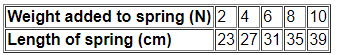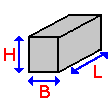# Forces and their effects quiz

#### Quiz Description

In this quiz, we will be looking at forces and their overall effects. It is important to know that, forces are used in our day to day life, we use forces to move a stationary object or the object at rest, stop the motion of an object, change the size and shape of the object, and also change the direction of motion of an object. For example, a footballer returning a kickback to another player or breaking a ball, in each of these situations, a force is used. This is one of the most essential topics in Physics, and a very common part in most Exams like GCE, GCSE, and even SATs.

This quiz has a lot of fascinating and exciting things. Especially when it comes to forces whose effects are so large in magnitude, like the Gravitational force. Some other forces whose effects are very noticeable are Magnetic and electrostatic forces. Along the line, you will know all the fundamental forces of nature.
Effects caused by forces are noticeable depending on how strong a force may be. These effects could be changing the direction of motion of an object, stopping an object from moving, or even deforming an object. For example, a force of magnitude like an engine force that causes motion can not compare with a gravitational force that holds the Earth in place. So effects of forces can be seen to mean what the force actually does when applied which can also be seen as consequences of applying a force.
These quizzes will be of much help to anyone preparing to write Exams or anyone looking for challenging questions. Take the quiz and Enjoy!!

You are given the following masses and volumes for various blocks of materials. From them choose which one has a density of 0.5 g/cm3?

Correct
•  material W: mass = 8 g, volume = 8 cm3
•  material X: mass = 8 g, volume = 16 cm3
•  material Z: mass = 16 g, volume = 8 cm3
•  material Y: mass = 12 g, volume = 8 cm3

Which word means the force of gravity on an object?

Correct
• friction
• weight
• pressure
• upthrust

Which of the following are units of weight?

Correct
• grams per centimetre cubed
• centimetre cubed
• kilogram
• newton

Which of the following is a 'force'?

Correct
• heat
• light
• gravity
• electricity

Which word means the force of attraction between any two objects?

Correct
• friction
• weight
• gravity
• upthrust

Which of the following are units of weight?

Correct
• N
• g/cm3
• kg
• cm3

Which of the following are units of mass?

Correct
• kilogram
• newton
• centimetre cubed
• grams per centimetre cubed

You are given the following masses and volumes for various blocks of materials. The mass of 1 cm3 of water is 1 g. From them choose which one will float on water?

Correct
•  material Y: mass = 30 g, volume = 28 cm3
•  material Z: mass = 12 g, volume = 13 cm3
•  material X: mass = 22 g, volume = 20 cm3
•  material W: mass = 12 g, volume = 10 cm3

Which of the following are units of density?

Correct
• kg
• N
• cm3
• g/cm3

Which of the following are units of density?

Correct
• kilogram
• grams per centimetre cubed
• centimetre cubed
• newton

Which of the following is a 'force'?

Correct
• heat
• kinetic
• buoyancy
• electricity

Which of the following is a 'force'?

Correct
• friction
• light
• electricity
• kinetic

Which of the following are units of speed?

Correct
• centimetre cubed
• newton
• kilogram
• metres per second

A student carried out an experiment by putting weights on the end of a spring. After each weight was added, the length of the spring was carefully measured. The results are summarised.

From the data, what is the real length of the spring with no weight on?Correct
• 19 cm
•  20 cm
• 21 cm
•  22 cm

Calculate the block volume if L = 15 cm, B = 2 cm and H = 4 cm.Correct
•   60 cm3
•  120 cm3
•  240 cm3
• 90 cm3